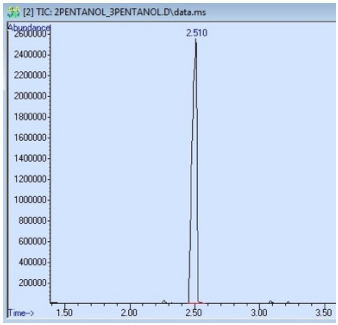# 2.5B: Uses of Gas Chromatography

•• Lisa Nichols
• Butte College
$$\newcommand{\vecs}{\overset { \rightharpoonup} {\mathbf{#1}} }$$ $$\newcommand{\vecd}{\overset{-\!-\!\rightharpoonup}{\vphantom{a}\smash {#1}}}$$$$\newcommand{\id}{\mathrm{id}}$$ $$\newcommand{\Span}{\mathrm{span}}$$ $$\newcommand{\kernel}{\mathrm{null}\,}$$ $$\newcommand{\range}{\mathrm{range}\,}$$ $$\newcommand{\RealPart}{\mathrm{Re}}$$ $$\newcommand{\ImaginaryPart}{\mathrm{Im}}$$ $$\newcommand{\Argument}{\mathrm{Arg}}$$ $$\newcommand{\norm}{\| #1 \|}$$ $$\newcommand{\inner}{\langle #1, #2 \rangle}$$ $$\newcommand{\Span}{\mathrm{span}}$$ $$\newcommand{\id}{\mathrm{id}}$$ $$\newcommand{\Span}{\mathrm{span}}$$ $$\newcommand{\kernel}{\mathrm{null}\,}$$ $$\newcommand{\range}{\mathrm{range}\,}$$ $$\newcommand{\RealPart}{\mathrm{Re}}$$ $$\newcommand{\ImaginaryPart}{\mathrm{Im}}$$ $$\newcommand{\Argument}{\mathrm{Arg}}$$ $$\newcommand{\norm}{\| #1 \|}$$ $$\newcommand{\inner}{\langle #1, #2 \rangle}$$ $$\newcommand{\Span}{\mathrm{span}}$$$$\newcommand{\AA}{\unicode[.8,0]{x212B}}$$

## Assessing Purity

A GC instrument is very good at verifying (or disproving) the purity of samples, and it can often spot trace quantities of impurity. In Figure 2.77, the GC spectra of "n-hexane" and "hexanes" are shown, two reagents that can often be found in a chemical stockroom. The GC spectrum of n-hexane (Figure 2.77b) shows one prominent peak, although there are hints of three other peaks on the baseline. The bottle of n-hexane claims it to be $$95\%$$ pure, which is consistent with what is seen in its GC spectrum. "Hexanes" however, is a true mixture, as there are multiple significant peaks in its GC spectrum (Figure 2.77d). Hexanes contains n-hexane as the major component, but also contains closely related isomers (2-methylpentane, 3-methylpentane, and methylcyclopentane).$$^{12}$$ Hexanes is a commonly used solvent, where its lack of purity is not crucial, and it is considerably cheaper than pure n-hexane.Figure 2.77: a) Bottle of n-hexane, b) GC spectrum of n-hexane, c) Bottle of "hexanes", d) GC spectrum of "hexanes". The compounds present in hexanes are: 2-methylpentane, 3-methylpentane, n-hexane, and methylcyclopentane (in order of elution).$$^{13}$$

A sample that produces a GC spectrum with only one peak is not always pure. In Figure 2.78 is the GC spectrum of a sample containing both 2-pentanol and 3-pentanol, even though only a single peak is observed. The GC column used for this run separates based on differences in boiling point, and these isomers differ in boiling point by only $$4^\text{o} \text{C}$$, causing them to co-elute.Figure 2.78: GC spectrum of a sample containing 2-pentanol and 3-pentanol. Both compounds are represented by the peak at $$2.510 \: \text{min}$$.

An impure sample can also produce a GC spectrum with only one peak if some components elute outside of the window of time detected by the instrument. For example, the GC spectrum in Figure 2.78 begins collecting data at $$1.50 \: \text{min}$$ (the value on the x-axis), and any compounds that elute before that time will not be detected. Components of a mixture may also not exit the column before the run is ceased, in which case they remain on the column and sometimes elute overtop of a subsequent run!

## Identifying Components

Due to the precision of retention times, GC can be used to identify components of a mixture if pure samples are acquired and if the components separate on the column.

For example, Figure 2.79a shows a GC spectrum of a sample containing both 3-pentanol and 1-pentanol. Boiling points can be used to predict which peak represents which isomer, but another definitive method is to run a GC of one of the pure components. Figure 2.79b shows a GC spectrum of 1-pentanol, and its retention time closely matches one of the two peaks in the original mixture, thus enabling its identification.Figure 2.79: a) GC spectrum of a sample containing 3-pentanol and 1-pentanol, b) GC spectrum of pure 1-pentanol.

Injection of known standards can be helpful in deciphering confusing mixtures, and for identifying closely eluting components, such as cis-trans isomers, where the difference in boiling points may be minimal. If using a mass spectrometer detector, a mass spectrum computer database is also helpful in identifying components.

$$^{12}$$Methylcyclopentane $$\left( \ce{C_6H_{12}} \right)$$ is not a true isomer of n-hexane $$\left( \ce{C_6H_{14}} \right)$$.

$$^{13}$$Complete removal of hexanes by rotary evaporation is sometimes difficult, and evidence of its persistence can be seen in $$\ce{^1H}$$ NMR samples by a doublet present around $$0.9 \: \text{ppm}$$ from the methyl of the methylcyclopentane impurity.

This page titled 2.5B: Uses of Gas Chromatography is shared under a CC BY-NC-ND 4.0 license and was authored, remixed, and/or curated by Lisa Nichols via source content that was edited to the style and standards of the LibreTexts platform; a detailed edit history is available upon request.